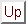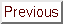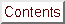Next: Non-Linear constraints Up: A short review of Previous: A short review of   Contents

Subsections

# Linear constraints

We want to find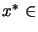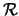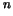which satisfies: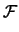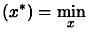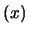Subject to: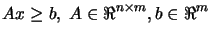(8.3)

whereis the dimension of the search space and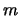is the number of linear constraints.

## Active set - Null space method

The material of this section is based on the following reference: [Fle87].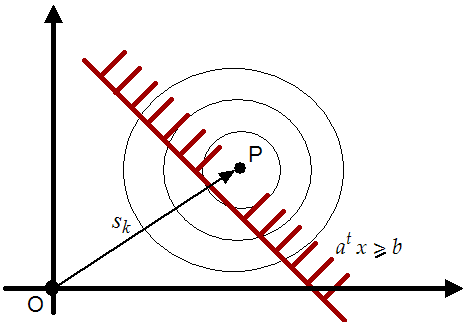At each step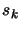computed from 8.1, we check if one of thelinear constraints has been violated. On the figure 8.1, the linear constraint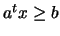has been violated and it's thus "activated". Without loosing generality, let's define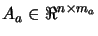and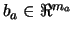the set of the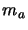active constraints.Letand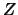be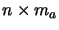and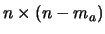matrices respectively such that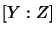is non-singular, and in addition let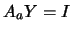and. The step must be feasible, so we must have to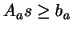. A solution to this equation is given by: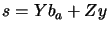where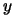can be any vector. Indeed, we have the following:. The situation is illustrated in figure 8.2. We will chooseas the solution of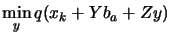subject to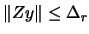(8.4)

with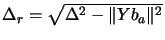. In other words,is the minimum of the quadratical approximation of the objective function limited to the reduced space of the active linear constraints and limited to the trust region boundaries. We have already developed an algorithm able to computein chapter 4.
When using this method, there is no difference between " approach 1" and "approach 2".
This algorithm is very stable in regards to rounding error. It's very fast because we can make Newton step (quadratical convergence speed) in the reduced space. Beside, we can use software developed in chapter 4. For all these reasons, it has been chosen and implemented. It will be fully described in chapter 9.

The material of this section is based on the following reference: [CGT00e].
In these methods, we will follow the "steepest descent steps": we will follow the gradient. When we enter the infeasible space, we will simply project the gradient into the feasible space. A straightforward (unfortunately false) extension to this technique is the "Newton step projection algorithm" which is illustrated in figure 8.3. In this figure the current point is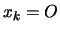. The Newton step () lead us to point P which is infeasible. We project P into the feasible space: we obtain B. Finally, we will thus follow the trajectory OAB, which seems good.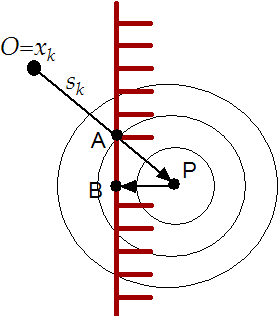In figure 8.4, we can see that the "Newton step projection algorithm" can lead to a false minimum. As before, we will follow the trajectory OAB. Unfortunately, the real minimum of the problem is C.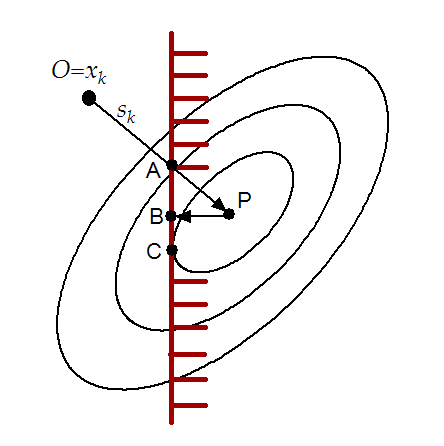We can therefore only follow the gradient,not the Newton step. The speed of this algorithm is thus, at most, linear, requiring many evaluation of the objective function. This has little consequences for "approach 1" but for "approach 2" it's intolerable. For these reasons, the Null-space method seems more promising and has been chosen.Next: Non-Linear constraints Up: A short review of Previous: A short review of   Contents
Frank Vanden Berghen 2004-04-19